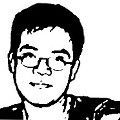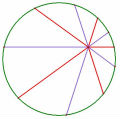# 97中興高中一題

## 97中興高中一題

a,b為自然數且a-b=2001,另外[a,b]=11339,則S={ax+by|x,y為整數}中之最小正數為(Ans:667)

TOP

 weiye 瑋岳發私訊 加為好友 目前離線 2# 大 中 小 發表於 2010-6-19 20:08  只看該作者 $$S=\left\{ax+by\Big|x,y \mbox{為整數}\right\}$$ 中之最小正數為 $$a,b$$ 之最大公因數 令 $$gcd(a,b)=d$$，且 $$a=dh, b=dk$$，其中 $$(h,k)=1$$ 則 $$d(h-k)=2001$$ 且 $$hkd=11339$$ 因為 $$(h,k)=1$$，所以 $$(hk, h-k)=1$$ 故，$$d=gcd(2001,11339)=667.$$ 註：此題目有誤，詳見後方回覆。感謝老王老師指正！^_^ UID1 帖子2170 閱讀權限200 上線時間8089 小時 註冊時間2006-3-5 最後登入2023-1-28  查看詳細資料 TOP
 老王 老王發私訊 加為好友 目前離線 3# 大 中 小 發表於 2010-6-19 20:22  只看該作者 我想，八神庵應該不是來亂問的 瑋岳老師，你的h,k各是多少?? 名豈文章著官應老病休飄飄何所似Essential isolated singularity UID261 帖子308 閱讀權限10 上線時間943 小時 註冊時間2009-5-14 最後登入2014-3-17  查看詳細資料 TOP
##### 引用:

（a-b=奇數，則 a, b 奇偶性不同，因此 [a,b] 必為偶數...矛盾）

TOP

 八神庵發私訊 加為好友 目前離線 5# 大 中 小 發表於 2010-6-19 21:08  只看該作者 這就是公佈教甄題目的秘奧義....debug 坦白說這題是97中興高中的題目 但我怎麼就是做不出來 感謝老王與瑋岳大精闢的找"茶".... UID523 帖子147 閱讀權限10 上線時間32 小時 註冊時間2010-5-15 最後登入2022-4-24  查看詳細資料 TOP
﻿